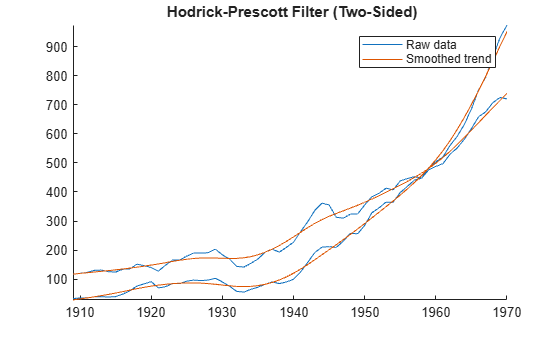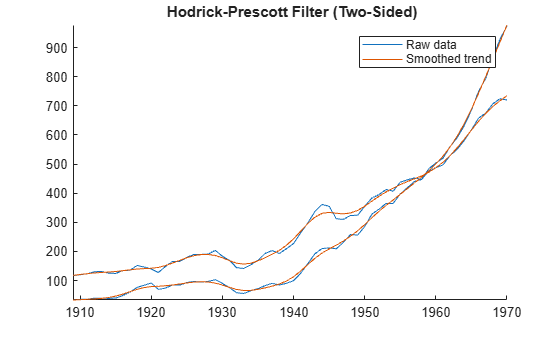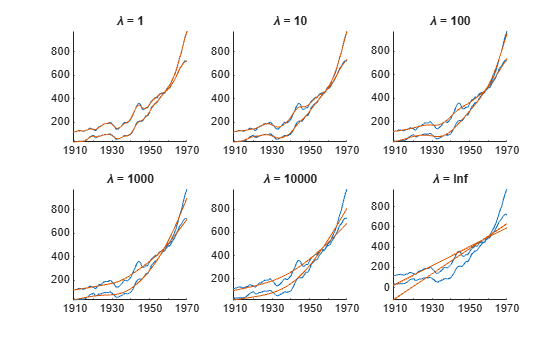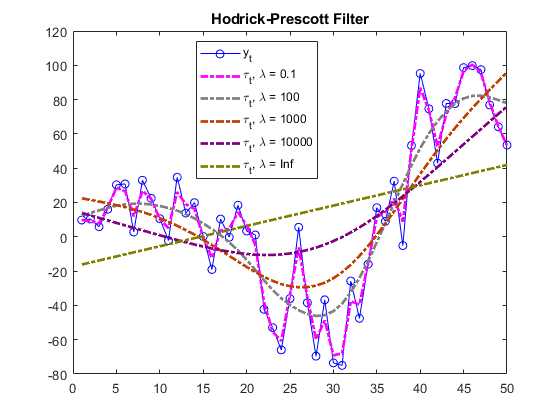# hpfilter

Hodrick-Prescott filter for trend and cyclical components

## Syntax

[Trend,Cyclical] = hpfilter(Y)
[TTbl,CTbl] = hpfilter(Tbl)
[___] = hpfilter(___,Name=Value)
hpfilter(___)
hpfilter(ax,___)
[___,h] = hpfilter(___)

## Description

Separate one or more time series into additive trend and cyclical components by applying the Hodrick-Prescott filter . hpfilter optionally plots the series and trend component, with cycles removed. The plot helps you select a smoothing parameter.

In addition to the Hodrick-Prescott filter, Econometrics Toolbox™ supports the Baxter-King (bkfilter), Christiano-Fitzgerald (cffilter), and Hamilton (hfilter) filters.

example

[Trend,Cyclical] = hpfilter(Y) returns the additive trend Trend and cyclical Cycilcal components from applying the Hodrick-Prescott filter to each variable (column) of the input matrix of time series data Y. The smoothing parameter default is 1600, suggested in  for quarterly data.

example

[TTbl,CTbl] = hpfilter(Tbl) returns the tables or timetables TTbl and CTbl containing variables for the trend and cyclical components, respectively, from applying the Hodrick-Prescott filter to each variable in the input table or timetable Tbl. To select different variables in Tbl to filter, use the DataVariables name-value argument. (since R2022a)

example

[___] = hpfilter(___,Name=Value) specifies options using one or more name-value arguments in addition to any of the input argument combinations in previous syntaxes. hpfilter returns the output argument combination for the corresponding input arguments. For example, hpfilter(Tbl,Smoothing=100,DataVariables=1:5) applies the Hodrick-Prescott filter to the first five variables in the input table Tbl and sets the smoothing parameter to 100. (since R2022a)

example

hpfilter(___) plots time series variables in the input data and their respective trend components, computed by the Hodrick-Prescott filter, on the same axes.
hpfilter(ax,___) plots on the axes specified by ax instead of the current axes (gca). ax can precede any of the input argument combinations in the previous syntaxes. (since R2022a)

example

[___,h] = hpfilter(___) plots the specified series and their trend components, and additionally returns handles to plotted graphics objects. Use elements of h to modify properties of the plot after you create it. (since R2022a)

## Examples

collapse all

Plot the cyclical component of the US post-WWII, seasonally adjusted, quarterly, real gross national product (GNPR).

load Data_GNP GNPR = Data(:,2); [trend,cyclical] = hpfilter(GNPR); T = numel(trend)
T = 235 

trend and cyclical are 235-by-1 vectors containing the trend and cyclical components, respectively, resulting from applying the Hodrick-Prescott filter to the series with default smoothing parameter 1600.

plot(dates,cyclical) axis tight ylabel("Real GNP Cyclical Component")Since R2022a

Apply the Hodrick-Prescott filter to all variables in input table variables.

Load the Schwert stock data set Data_SchwertStock.mat, which contains monthly returns of the NYSE index from 1871 through 2008 in DataTimeTableMth, among three other variables (for details, enter Description). Remove all missing observations from all series.

load Data_SchwertStock TTM = rmmissing(DataTimeTableMth);

Aggregate the monthly data in the timetable to quarterly measurements.

TTQ = convert2quarterly(TTM);

Apply the Hodrick-Prescott filter to all variables in the quarterly timetable. The default smoothing parameter value is 1600. Display the last few observed components.

[TQTT,CQTT] = hpfilter(TTQ); size(TQTT)
ans = 1×2 220 4 
tail(TQTT)
 Time Return DivYld CapGain CapGainA ___________ _________ _________ ___________ ___________ 31-Mar-1924 0.0046179 0.0067678 -0.0021499 -0.0021499 30-Jun-1924 0.005586 0.0067204 -0.0011344 -0.0011344 30-Sep-1924 0.0066431 0.0066689 -2.5812e-05 -2.5812e-05 31-Dec-1924 0.0077772 0.0066142 0.0011629 0.0011629 31-Mar-1925 0.0089687 0.0065574 0.0024113 0.0024113 30-Jun-1925 0.010231 0.0064998 0.0037314 0.0037314 30-Sep-1925 0.011543 0.0064423 0.0051005 0.0051005 31-Dec-1925 0.012878 0.0063854 0.0064922 0.0064922 
tail(CQTT)
 Time Return DivYld CapGain CapGainA ___________ __________ ___________ __________ __________ 31-Mar-1924 -0.019683 0.00033141 -0.020014 -0.020014 30-Jun-1924 0.059151 5.7125e-05 0.059093 0.059093 30-Sep-1924 -0.011788 0.00044598 -0.012234 -0.012234 31-Dec-1924 0.052846 0.00052627 0.05232 0.05232 31-Mar-1925 -0.056652 -0.00078018 -0.055872 -0.055872 30-Jun-1925 -0.0069909 -0.0010415 -0.0059494 -0.0059494 30-Sep-1925 0.0052352 -0.0011402 0.0063755 0.0063755 31-Dec-1925 0.036713 0.00070754 0.036006 0.036006 

TQTT and CQTT are 220-by-4 timetables containing the trend and cyclical components, respectively, of the series in TTQ. Variables in the input and output timetables correspond.

By default, hpfilter filters all variables in the input table or timetable. To select a subset of variables, set the DataVariables option.

To compare outputs between different tabular inputs, apply the Hodrick-Prescott filter to all variables in the table of monthly data DataTableMth and the timetable of monthly data TTM.

% Table input of daily data DTM = rmmissing(DataTableMth); [TMDT,CMDT] = hpfilter(DTM); size(TMDT)
ans = 1×2 656 4 
tail(TMDT)
 Return DivYld CapGain CapGainA ________ _________ ________ ________ May1925 0.02665 0.0045709 0.022079 0.022079 Jun1925 0.0275 0.004558 0.022942 0.022942 Jul1925 0.028372 0.0045491 0.023823 0.023823 Aug1925 0.02926 0.0045437 0.024716 0.024716 Sep1925 0.030152 0.004542 0.02561 0.02561 Oct1925 0.031049 0.004543 0.026506 0.026506 Nov1925 0.031941 0.0045461 0.027395 0.027395 Dec1925 0.032844 0.0045508 0.028293 0.028293 
tail(CMDT)
 Return DivYld CapGain CapGainA __________ __________ _________ _________ May1925 0.039536 -0.0020878 0.041624 0.041624 Jun1925 -0.02426 0.00090028 -0.02516 -0.02516 Jul1925 -0.0095168 0.00066325 -0.01018 -0.01018 Aug1925 0.018948 -0.0018318 0.02078 0.02078 Sep1925 -0.013374 0.00076008 -0.014134 -0.014134 Oct1925 0.037148 9.6588e-05 0.037051 0.037051 Nov1925 -0.040405 -0.0017316 -0.038674 -0.038674 Dec1925 0.016747 0.0025421 0.014205 0.014205 
% Timetable input of daily data [TMTT,CMTT] = hpfilter(TTM); size(TMTT)
ans = 1×2 656 4 
tail(TMTT)
 Time Return DivYld CapGain CapGainA ___________ ________ _________ ________ ________ 01-May-1925 0.02665 0.0045709 0.022079 0.022079 01-Jun-1925 0.0275 0.004558 0.022942 0.022942 01-Jul-1925 0.028372 0.0045491 0.023823 0.023823 01-Aug-1925 0.02926 0.0045437 0.024716 0.024716 01-Sep-1925 0.030152 0.004542 0.02561 0.02561 01-Oct-1925 0.031049 0.004543 0.026506 0.026506 01-Nov-1925 0.031941 0.0045461 0.027395 0.027395 01-Dec-1925 0.032844 0.0045508 0.028293 0.028293 
tail(CMTT)
 Time Return DivYld CapGain CapGainA ___________ __________ __________ _________ _________ 01-May-1925 0.039536 -0.0020878 0.041624 0.041624 01-Jun-1925 -0.02426 0.00090028 -0.02516 -0.02516 01-Jul-1925 -0.0095168 0.00066325 -0.01018 -0.01018 01-Aug-1925 0.018948 -0.0018318 0.02078 0.02078 01-Sep-1925 -0.013374 0.00076008 -0.014134 -0.014134 01-Oct-1925 0.037148 9.6588e-05 0.037051 0.037051 01-Nov-1925 -0.040405 -0.0017316 -0.038674 -0.038674 01-Dec-1925 0.016747 0.0025421 0.014205 0.014205 

Because the data is disaggregated, the outputs of the daily data have more rows than from the quarterly data. The filter results of the daily inputs are equal among the corresponding outputs, but hpfilter returns tables of results, instead of timetables, when you supply data in a table.

Since R2022a

Load the Nelson-Plosser macroeconomic data set Data_NelsonPlosser.mat, which contains series measured yearly in the table DataTable.

load Data_NelsonPlosser

Filter the real and nominal GNP series, GNPR and GNPN, respectively. Plot the trend component with each series by additionally returning the vector of graphics objects. Set the smoothing parameter to 100, as suggested in  for yearly data.

[TTblHP,CTblHP,hHP] = hpfilter(DataTimeTable,Smoothing=100, ... DataVariables=["GNPR" "GNPN"]);Filter the series again, but set the smoothing parameter to 6.25, which is the suggested smoothing parameter value in  for yearly data.

figure [TTblRU,CTblRU,hRU] = hpfilter(DataTimeTable,Smoothing=6.25, ... DataVariables=["GNPR" "GNPN"]);The smoothed trend of the latter plot contains more higher frequency cycles than the former plot, and, therefore, the smoothed trend in the latter plot follows the data more tightly.

Experiment with the smoothing parameter value by filtering the series several more times and setting the smoothing parameter to 0, 10, 100, 1000, 10,000, and Inf. Plot each set of results by not returning any outputs.

smoothing = [10.^(0:4) Inf]; tiledlayout(2,3) for j = 1:numel(smoothing) nexttile hpfilter(DataTimeTable,Smoothing=smoothing(j), ... DataVariables=["GNPR" "GNPN"]); title("\lambda = " + string(smoothing(j))); legend("off") end## Input Arguments

collapse all

Time series data, specified as a numObs-by-numVars numeric matrix. Each column of Y corresponds to a variable, and each row corresponds to an observation.

Data Types: double

Since R2022a

Time series data, specified as a table or timetable with numObs rows. Each row of Tbl is an observation.

Specify numVars variables to filter by using the DataVariables argument. The selected variables must be numeric.

Since R2022a

Axes on which to plot, specified as an Axes object.

By default, hpfilter plots to the current axes (gca).

Note

hpfilter removes, from the specified data, all rows containing at least one missing observation, represented by a NaN value.

### Name-Value Arguments

Specify optional pairs of arguments as Name1=Value1,...,NameN=ValueN, where Name is the argument name and Value is the corresponding value. Name-value arguments must appear after other arguments, but the order of the pairs does not matter.

Before R2021a, use commas to separate each name and value, and enclose Name in quotes.

Example: hpfilter(Tbl,Smoothing=100,DataVariables=1:5) applies the Hodrick-Prescott filter to the first five variables in the input table Tbl and sets the smoothing parameter to 100.

Since R2022a

Trend component smoothing parameter, specified as a nonnegative numeric scalar or vector of length numVars.

For a scalar, hpfilter applies Smoothing to all specified input series. For a vector, hpfilter applies Smoothing(k) to specified input series k in the data.

If Smoothing(k) = 0, hpfilter does not smooth the corresponding trend components. In this case, these conditions apply:

• Trend(:,k) = yk, where yk is the input data of specified variable k.

• Cyclical(:,k) = zeros(numObs,1).

If Smoothing(k) = Inf, hpfilter applies maximum smoothing. In this case, these conditions apply:

• Trend(:,k) is the linear time trend computed by least squares.

• Cyclical(:,k) is the detrended series.

As the smoothing parameter increases, Trend approaches the linear time trend.

The default is 1600, which is suggested in  and  for quarterly data.

Example: Smoothing=100

Data Types: double

Since R2023a

Type of filter, specified as value in this table.

ValueDescription
"two-sided"hpfilter implements the two-sided Hodrick-Prescott filter .
"one-sided"hpfilter implements the one-sided Hodrick-Prescott filter .

Example: FilterType="one-sided"

Data Types: char | string

Since R2022a

Variables in Tbl that hpfilter filters, specified as a string vector or cell vector of character vectors containing variable names in Tbl.Properties.VariableNames, or an integer or logical vector representing the indices of names. The selected variables must be numeric.

Example: DataVariables=["GDP" "CPI"]

Example: DataVariables=[true true false false] or DataVariables=[1 2] selects the first and second table variables.

Data Types: double | logical | char | cell | string

## Output Arguments

collapse all

Trend component τt of each series in the data, returned as a numObs-by-numVars numeric matrix. hpfilter returns Trend when you supply the input Y.

Cyclical component ct of each series in the data, returned as a numObs-by-numVars numeric matrix. hpfilter returns Cyclical when you supply the input Y.

Since R2022a

Trend component τt of each specified series, returned as a numObs-by-numVars table or timetable, the same data type as Tbl. hpfilter returns TTbl when you supply the input Tbl.

Since R2022a

Cyclical component ct of each specified series, returned as a numObs-by-numVars table or timetable, the same data type as Tbl. hpfilter returns CTbl when you supply the input Tbl.

Since R2022a

Handles to plotted graphics objects, returned as a vector of graphics objects. hpfilter plots the data and trend only when you return no outputs or you return h.

h contains unique plot identifiers, which you can use to query or modify properties of the plot.

collapse all

### Hodrick-Prescott Filter

The Hodrick-Prescott filter decomposes an observed time series yt (Y) into a trend component τt (Trend) and a cyclical component ct (Cyclical) such that yt = τt + ct. The method implements a high-pass filter for the cycle that penalizes variations in the trend to a degree determined by the smoothing parameter .

The objective function of the filter is

$f\left({\tau }_{t}\right)=\sum _{t=1}^{T}{\left({y}_{t}-{\tau }_{t}\right)}^{2}+\lambda \sum _{t=2}^{T-1}{\left[\left({\tau }_{t+1}-{\tau }_{t}\right)-\left({\tau }_{t}-{\tau }_{t-1}\right)\right]}^{2},$

where:

The programming problem is to minimize the objective function over τ1,…,τT. The objective penalizes the sum of squares for the cyclical component with the sum of squares of second-order differences for the trend component (trend acceleration penalty). If λ = 0, the minimum of the objective is 0 with τt = yt for all t. As λ increases, the penalty for a flexible trend increases, resulting in an increasingly smoother trend. When λ is arbitrarily large, the trend acceleration approaches 0, resulting in a linear trend.

This figure shows the effects of increasing the smoothing parameter on the trend component for a simulated series.The filter is equivalent to a cubic spline smoother, where the smoothed component is τt.

## Tips

• Hodrick and Prescott  suggest values for the smoothing parameter λ (Smoothing) that depend upon the periodicity of the data, and Ravn and Uhlig  suggest adjustments to those values. This table contains their suggested smoothing parameter values for several data periodicities.

PeriodicityHodrick and Prescott Suggested SmoothingRavn and Uhlig Suggested Smoothing
Yearly1006.25
Quarterly16001600
Monthly14400129600

• Supply a vector of smoothing parameters for the Smoothing name-value argument to test alternatives. Plot the results to visually compare the alternatives.

• The default two-sided filter (see FilterType) uses future values of the input series to compute outputs at time t. Because the filter is typically applied to historical data, the results can contain anomalous end effects unsuitable for forecasting . The one-sided filter, by contrast, is causal because it uses only current and previous values of the input series. As a result, the one-sided filter does not revise outputs when new data becomes available.

 Hodrick, Robert J., and Edward C. Prescott. "Postwar U.S. Business Cycles: An Empirical Investigation." Journal of Money, Credit and Banking 29, no. 1 (February 1997): 1–16. https://doi.org/10.2307/2953682.

 Hodrick, Robert J. "An Exploration of Trend-Cycle Decomposition Methodologies in Simulated Data." National Bureau of Economic Research Working Paper No. w26750. Social Science Research Network (February 2020). https://papers.ssrn.com/abstract=3539317.

 Ravn, Morton O., and Harald Uhlig. "On Adjusting the Hodrick-Prescott Filter for the Frequency of Observations." The Review of Economics and Statistics 84 , no. 2 (May 2002): 371–76. https://doi.org/10.1162/003465302317411604.

 Stock, James H., and Mark W. Watson. "Forecasting Inflation." Journal of Monetary Economics 44, no. 2 (October 1999): 293–335. https://doi.org/10.1016/S0304-3932(99)00027-6.